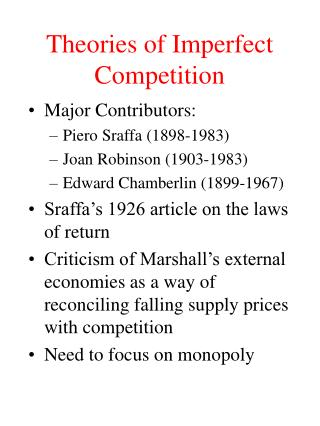# Theories of Imperfect Competition - PowerPoint PPT PresentationDownload PresentationTheories of Imperfect Competition

Theories of Imperfect CompetitionDownload Presentation## Theories of Imperfect Competition

- - - - - - - - - - - - - - - - - - - - - - - - - - - E N D - - - - - - - - - - - - - - - - - - - - - - - - - - -
##### Presentation Transcript

1. Theories of Imperfect Competition • Major Contributors: • Piero Sraffa (1898-1983) • Joan Robinson (1903-1983) • Edward Chamberlin (1899-1967) • Sraffa’s 1926 article on the laws of return • Criticism of Marshall’s external economies as a way of reconciling falling supply prices with competition • Need to focus on monopoly

2. Joan Robinson and Imperfect Competition • The Economics of Imperfect Competition (1933) • Introduction of marginal revenue curves • Deals with an individual firm assuming the firm has its own market and faces a downward sloping demand curve • In the absence of new entry, the analysis is as for a monopoly

3. Monopoly Equilibrium • A monopoly faces the market demand curve • For a single price monopoly the D curve is the AR curve • MR will lie below AR curve • Monopoly profit max equilibrium where MC=MR • Second order condition is that the MC cuts the MR from below

4. Monopoly Equilibrium MC P D=AR MR Q Point a is not an equilibrium P MC a b D=AR MR Q

5. Monopoly Equilibrium • Firm will have excess profits if P > ATC • If no new entry of other firms selling substitute goods excess profit can remain • Idea of “full equilibrium” where other firms come in and all firms are where MC =MR and P = ATC but each firm still facing a downward sloping demand curve

6. Price Discrimination • Perfect price discrimination • D curve becomes the MR curve • No restriction of output P MC Total revenue D=MR Q Q

7. Price Discrimination • Market segmentation • Profit max output where the aggregate MR=MC • Allocate output between markets so as to equalize MR \$ MC MR MR1+MR2 Q Total Q

8. Price Discrimination p1 D1 p2 MR D2 MR1 MR2 q2 q1 Price discrimination of this type may or may not increase total output as compared with a single price monopolist depending on exact shapes of the demand curves. In the case of linear demand curves total output will be the same

9. Imperfect Factor Markets • Effects of monopoly in output market on the factor market • Firms will hire where W=MRP • But MRP<VMP • Monopoly exploitation of labour Wage S w D comp D monop L l

10. Imperfect Factor Markets • Effects of monopsony in the factor market • Single buyer in the labour market • Faces upward sloping supply curve for the factor • Marginal cost of the factor lies above the supply curve • Firm equates MRP with MC of the factor • Wage below VMP • Monopsony exploitation of labour

11. Monopsony Exploitation W MC of labour S mrp w D=MRP L l Difference between mrp and w is monopsony exploitation of labour

12. Edward Chamberlin: Monopolistic Competition • Theory of Monopolistic Competition 1933 • Very different starting point from Robinson • Not an issue with Marshall’s laws of return, but a response to the existence of advertising and product differentiation • Firms have monopoly over their own brands but there are many close substitutes

13. Monopolistic Competition: Demand • Firms face two demand curves • one showing the demand with the prices of other brands given (dd curve) • the other is a share of the market curve which is drawn for this brand assuming all brands have the same price (DD curve) • Chamberlin assumes symmetry between firms

14. Monopolistic Competition Demand curves facing the firm P D d p d D q Q

15. Monopolistic Competition • Monopolistic competition • Large group and small group models • Large group: like perfect competition but for product differentiation • Small group: oligopoly, barriers to entry: like monopoly but an issue of firms being aware of their interdependence

16. Monopolistic Competition: Large Group • Equilibrium for the individual firm is where mr (derived from the dd curve) = MC • For this to be consistent with equilibrium for the group the firm must also be on its share of the market demand curve • In the long run all firms must just be making normal profits due to free entry condition • Long run equilibrium will be to the lest of min LRACT

17. Large Group Equilibrium P Short Run D d MC p d mr D q Q P Long run D MC d LRATC p d mr D Q q

18. Small Group Model • Small number of firms • Barriers to entry • If all firms charge the same price then each firm only faces the DD demand curve • Similar to monopoly equilibrium P D p MC D MR Q q

19. Kinked Demand Curve Model • But will all firms charge the same price? What happens if one firm changes price? • That firm might believe that other firms will follow price cuts but will not follow price rises • Paul Sweezy and the kinked demand curve model (1939) • Discontinuity in MR curve • Price inflexibility thesis

20. Kinked Demand Curve Model P D d p d D Q q P MC’ P MC” D MR Q

21. General Problem of Oligopoly Analysis • Problem of interdependence • Cournot model of duopoly • Stackelberg and price leadership models • More recent game theory approaches– oligopoly as a prisoners’ dilemma game • Cournot-Nash equilibrium • One shot and repeated games • Evolutionary game theory and evolutionary stable strategies